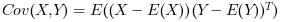Scilab Home page | Wiki | Bug tracker | Forge | Mailing list archives | ATOMS | File exchange
Change language to: English - Français - Português - 日本語 -

See the recommended documentation of this function

# cov

Covariance matrix

### Calling Sequence

```C = cov(x)
C = cov(x, 0)
C = cov(x, 1)
C = cov(x, y)
C = cov(x, y, 0)
C = cov(x, y, 1)```

### Parameters

x

a nobs-by-1 or nobs-by-nvar matrix of doubles

y

a nobs-by-1 or nobs-by-nvar matrix of doubles

C

a square matrix of doubles, the empirical covariance

### Description

If x is a nobs-by-1 matrix, then `cov(x)` returns the variance of x, normalized by nobs-1.

If x is a nobs-by-nvar matrix, then `cov(x)` returns the nvar-by-nvar covariance matrix of the columns of x, normalized by nobs-1. Here, each column of x is a variable and each row of x is an observation.

If x and y are two nobs-by-1 matrices, then `cov(x, y)` returns the 2-by-2 covariance matrix of x and y, normalized by nobs-1, where nobs is the number of observations.

`cov(x, 0)` is the same as `cov(x)` and `cov(x, y, 0)` is the same as `cov(x, y)`. In this case, if the population is from a normal distribution, then C is the best unbiased estimate of the covariance matrix.

`cov(x, 1)` and `cov(x, y, 1)` normalize by nobs. In this case, C is the second moment matrix of the observations about their mean.

The covariance of X and Y is defined by:where E is the expectation.

This function is compatible with Matlab.

### Examples

```x = [1; 2];
y = [3; 4];
C = cov(x, y)
expected = [0.5, 0.5; 0.5, 0.5];
C = cov([x, y])```
```x = [230; 181; 165; 150; 97; 192; 181; 189; 172; 170];
y = [125; 99; 97; 115; 120; 100; 80; 90; 95; 125];
expected = [
1152.4556, -88.911111
-88.911111, 244.26667
];
C = cov(x, y)
C = cov([x, y])```
```// Source 
A = [
4.0 2.0 0.60
4.2 2.1 0.59
3.9 2.0 0.58
4.3 2.1 0.62
4.1 2.2 0.63
];
S = [
0.025 0.0075 0.00175
0.0075 0.007 0.00135
0.00175 0.00135 0.00043
];
C = cov(A)```

### Bibliography

Wikipedia: Covariance matrix

"Introduction to probability and statistics for engineers and scientists", Sheldon Ross

### History

 Версия Описание 5.5.0 cov function added, to improve mvvacov (deprecated)